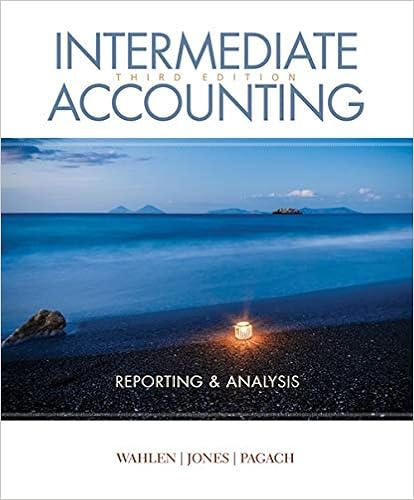# W 455 461 gl 74 269 271 dollar value lifo 30 use of

• Notes
• 10

This preview shows page 8 - 9 out of 10 pages.

##### We have textbook solutions for you!
The document you are viewing contains questions related to this textbook.The document you are viewing contains questions related to this textbook.
Chapter 7 / Exercise 7-21
Intermediate Accounting: Reporting and Analysis
Jones/WahlenExpert Verified
(W 455-461; GL 7.4, 269-271)
30. Use of the specific-goods pooled approach can result in problems for companies that often change the mix of their products, materials, and production methods
31. To compute inventory under the Dollar-Value LIFO Method a. New inventory layers (pools) are formed when the ending inventory (stated in base-year costs) exceeds the base-year costs of Beginning Inventory b. Calculation of Dollar-Value LIFO1) Determine the value of Ending Inventory at the most current cost (Similar to FIFO) 2) Divide by the current Price Indexa) This restates current cost of Ending Inventory to base-year cost (price prevailing when LIFO was adopted) b) Some companies can use published externalprice indices c)Some companies must compute internalprice indices, using the Double-Extension Method i. The Price Index for the current year = Value of Ending Inventory for the Period (at Current-Year Costs) Value of Ending Inventory for the Period (at Base-Year Costs) ii. The Price index is stated as a (number + decimal), such as 1.25 or 2.5 3) Split the Ending Inventory (by quantity) into layers, based on the year the items were acquired 4) Multiply each layer by the appropriate Price Index (price index for the year of acquisition) to obtain Ending Inventory at Dollar-Value LIFO cost a) Increases in Ending Inventory (at base-year cost) over Beg. Inventory (at base-year cost) are priced at current cost b) If Ending Inventory (at base-year costs) is less than Beginning Inventory (at base-year cost), the decrease is subtracted from the most recently added layer c. The Dollar-Value Method is a more practical way of valuing a complex, multiple-item inventory than the traditional LIFO method d. Illustration 8-5 shows dollar-value LIFO computations. e. Under certain circumstances the Dollar-Value LIFO approach produces the same Ending Inventory value as the Specific Goods LIFO approach (Illustration 8-6 demonstrates this) Accounting Required: 1. Calculate Ending Inventory, COGS, & Gross Profit using Dollar-Value LIFO 2. Calculate a Price Index, given various inputs Exercises: BE8-8, BE8-9, E8-22 (Part b), E8-23(Price Index), E8-24, E8-25, E8-26, P8-8, P8-9 (EI & Price Index), P8-10 (EI & Price Index), P8-11, GL 7.4
##### We have textbook solutions for you!
The document you are viewing contains questions related to this textbook.The document you are viewing contains questions related to this textbook.
Chapter 7 / Exercise 7-21
Intermediate Accounting: Reporting and Analysis
Jones/WahlenExpert Verified
•••